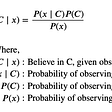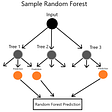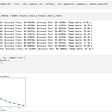# Linear Regression From Scratch With Python

## Implementing one of the most basic concepts in Data Science

As one of the most basic concepts in Data Science, I thought that it would be a good idea to cover the fundamentals of how linear regression works.

Here’s a link to the Github Repo:

# What Is Linear Regression?

Linear regression is a method for approximating a linear relationship between two variables. While that may sound complicated, all it really means is that it takes some input variable, like the age of a house, and finds out how it’s related to another variable, for example, the price it sells at.

Linear regression takes some input variable, like the age of a house, and finds out how it’s related to another variable, for example, the price it sells at.

We use it when the data has a linear relationship, which means that when you plot the points on a graph, the data lies approximately in the shape of a straight line.

The goal of linear regression is to find a line that best fits a set of data points.

In terms of general intuition, linear regression guesses a line that fits the data, sees how incorrect it was, and then adjusts itself to become slightly more accurate. It repeats this process until it’s reduced the error as much as possible.

Linear Regression involves a couple of steps:

1. Randomly initializing parameters for the hypothesis function
2. Computing the mean squared error
3. Calculating the partial derivatives
4. Updating the parameters based on the derivatives and the learning rate
5. Repeating from 2–4 until the error is minimized

While these may sound complicated, let’s go through them step by step and understand what each of them means.

# The Data

For linear regression, we need a dataset that follows a linear pattern to train our model on.

The python package sklearn comes with an inbuilt function to create a linear dataset:

# The Hypothesis Function

The linear equation is the standard form that represents a straight line on a graph, where m represents the gradient- how steep the line is, and b represents the y-intercept- where the line crosses the y-axis.

You might remember this as one of the first equations you learned in school.

The Hypothesis Function is the exact same function in the notation of Linear Regression.

The two variables we can change — m and b — are represented as parameters θ₁ and θ₀.

We’ll represent the function in python like so:

In the beginning, we randomly initialize our parameters, which means we give θ₁ and θ₀ random values to begin with. This will output a random line, maybe something like this:

We can create a function in python that displays the graph with the line using the matplotlib and numpy libraries:

Then, we can display a line with randomly initialized θ₁ and θ₀ values

When we run that function, it’ll output a random line, maybe something like this:

# The Error Function

Clearly, the line drawn in the graph above is wrong. But how wrong is it? That’s what the error function is for — it calculates the total error of your line. We’ll be using an error function called the Mean Squared Error function, or MSE, represented by the letter J.

Now while that may look complicated, what it’s doing is actually quite simple.

1. To find out how “wrong” the line is, we need to find out how far it is from each point. To do this, we subtract the actual value yᵢ from the predicted value h(xᵢ).
2. However, we don’t want the error to be negative, so to make sure it’s positive at all times, we square this value.
3. M is the number of points in our dataset. We then repeat this subtraction and squaring for all m points
4. Finally, we divide the error by 2. This will help us later when we are updating our parameters.

Here’s what that looks like in code:

Now that we have a value for how wrong our function is, we need to adjust the function to reduce this error.

# Calculating Derivatives

Our goal with linear regression was to find the line which best fits a set of data points. In other words, it’s the line that’s the least incorrect or has the lowest error.

If we graph our parameters against the error (i.e graphing the cost function), we’ll find that it forms something similar to the graph below. At the lowest point of that graph, the error is at it’s lowest. Finding this point is called minimizing the cost function.

To do this, we need to consider what happens at the bottom of the graph — the gradient is zero. So to minimize the cost function, we need to get the gradient to zero.

The gradient is given by the derivative of the function, and the partial derivatives of the functions are:

We can calculate the derivatives using the following function

# Updating The Parameters Based On The Learning Rate

Now we need to update our parameters to reduce the gradient. To do this, we use the gradient update rule

Alpha (α) is what we call the Learning rate, which is a small number that allows the parameters to be updated by a small amount. As mentioned above, we are trying to update the gradient such that it’s closer to zero (the bottom). The learning rate helps guide the network to the lowest point on the curve by small amounts.

# Minimizing the Cost Function

Now we repeat these steps — checking the error, calculating the derivatives, and updating the weights until the error is as low as possible. This is called minimizing the cost function.

We can now tie all our code together in a function in python:

When you run this, it randomly initializes θ₁ and θ₀, and then iterates 1000 times to update the parameters to reduce the error. Every 100 times, it outputs what the line looks like to show us our progress.

Once your error is minimized, your line should now be the best possible fit to approximate the data!

# Conclusion

Here are some key terms we learned —

Simple linear regression — Finds the relationship between two variables that are linearly correlated. E.g. finding the relationship between the size of a house and the price of a house

Linear relationship — When you plot the dataset on a graph, the data lies approximately in the shape of a straight line.

Linear equation — y = mx +b. The standard form that represents a straight line on a graph, where m represents the gradient, and b represents the y-intercept.

Gradient — How steep the line is

Y-intercept — Where the line crosses the y-axis

Random Initialization — Giving parameters random values to begin with

Cost function — Calculates the total error of your line

Minimizing the cost function — Reducing the value of the cost function until the error is minimized.

Learning rate (alpha (α)) — A small number that allows the parameters to be updated by a tiny amount.

After reading this article, I hope you now have a better understanding of linear regression and the gradient update rule, which is foundational for other concepts in the field.

Sarvasv

--

--

--

## More from Sigmoid

Making Machine Learning more accessible. One line of code at a time.

## Rowing Splits vs. Workout Duration## Introduction to Image Classification via Neural Networks: A Beginner’s Guide## Simple Cohort Analysis: A Powerful Way to Uncover Unique Behaviors## Citizen Data Scientist vs. Data Scientist: What’s the Difference?## Easy Python Code for Image Data Augmentation## Insights into food access through data visualizations## Sarvasv Kulpati

Writing about technology, philosophy, and everything in between.

## Implementing Naïve Bayes Classification from Scratch with Python## 3 ways to create a Deep Neural Network model with Keras and Tensorflow 2.0## Imagine Recognition Through Supervised Learning in Python## MNIST from Scratch